Documentation

### This is machine translation

Mouseover text to see original. Click the button below to return to the English version of the page.

## Choose a Solver

To simulate a dynamic system, you compute its states at successive time steps over a specified time span. This computation uses information provided by a model of the system. Time steps are time intervals when the computation happens. The size of this time interval is called step size. The process of computing the states of a model in this manner is known as solving the model. No single method of solving a model applies to all systems. Simulink® provides a set of programs called solvers. Each solver embodies a particular approach to solving a model.

A solver applies a numerical method to solve the set of ordinary differential equations that represent the model. Through this computation, it determines the time of the next simulation step. In the process of solving this initial value problem, the solver also satisfies the accuracy requirements that you specify.

Simulink provides two main types of solvers —fixed-step and variable-step solvers. You can see the solvers under each type in the Solver pane of model configuration parameters.

### Solver Types

Solvers are broadly classified using these criteria:

• The type of step size used in the computation

• Fixed-step solvers, as the name suggests, solve the model at fixed step sizes from the beginning to the end of the simulation. You can specify the step size or let the solver choose it. Generally, decreasing the step size increases the accuracy of the results and increases the time required to simulate the system.

• Variable-step solvers vary the step size during the simulation. They reduce the step size to increase accuracy when the states of a model change rapidly and during zero-crossing events. They increase the step size to avoid taking unnecessary steps when the states of a model change slowly. Computing the step size adds to the computational overhead at each step. However, it can reduce the total number of steps, and hence the simulation time required to maintain a specified level of accuracy for models with piecewise continuous or rapidly changing states.

• The nature of states in the model

• Continuous solvers use numerical integration to compute continuous states of a model at the current time step based on the states at previous time steps and the state derivatives. Continuous solvers rely on individual blocks to compute the values of the discrete states of the model at each time step.

• Discrete solvers are primarily for solving purely discrete models. They compute only the next simulation time step for a model. When they perform this computation, they rely on each block in the model to update its individual discrete state. They do not compute continuous states.

### Compare Solvers

When choosing a solver for simulating a model, consider:

• The dynamics of the system

• The stability of the solution

• The speed of computation

• The robustness of the solver

A solver might not completely satisfy all of your requirements, so use an iterative approach when choosing one. Compare simulation results from several solvers and select one that offers the best performance with minimal tradeoffs.

There are two ways to select a solver for your model:

• Use auto solver. New models have their solver selection set to auto solver by default. Auto solver recommends a fixed-step or variable-step solver for your model as well as maximum step size. For more information, see Use Auto Solver to Select a Solver

• If you are not satisfied with simulation results using auto solver, select a solver in the Solver pane in the model configuration parameters.

When you build and simulate a model, you can choose the solver based on the dynamics of your model. A variable-step solver is better suited for purely continuous models, like the dynamics of a mass spring damper system. A fixed-step solver is recommended for a model that contains several switches, like an inverter power system, due to the number of solver resets that would cause a variable-step solver to behave like a fixed-step solver.When you deploy a model as generated code, you can use only a fixed-step solver. If you select a variable-step solver during simulation, use it to calculate the step size required for the fixed-step solver that you need at deployment.

This chart provides a broad classification of solvers in the Simulink library.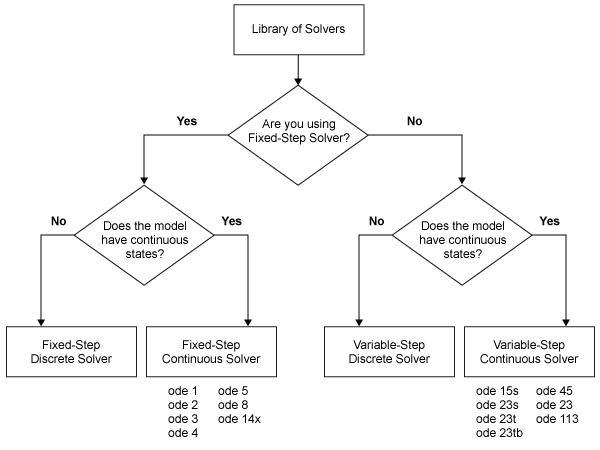To tailor the selected solver to your model, see Check and Improve Simulation Accuracy.

Ideally, the solver you select should:

• Solve the model successfully.

• For variable-step solvers, provide a solution within the tolerance limits you specify.

• Solve the model in a reasonable duration.

A single solver might not meet all of these requirements. Try simulating with several solvers before making a selection.

The Simulink library provides several solvers, all of which can work with the algebraic loop solver. For more information, see How the Algebraic Loop Solver Works.

In the Solver pane of model configuration parameters, the Simulink library of solvers is divided into two major types. See Fixed-Step Versus Variable-Step Solvers.

You can further categorize the solvers of each type:

• Discrete Versus Continuous Solvers

• Explicit Versus Implicit Continuous Solvers

• One Step Versus Multistep Continuous Solvers

• Single Order Versus Variable Order Continuous Solvers

#### Fixed-Step Versus Variable-Step Solvers

Fixed-step and variable-step solvers compute the next simulation time as the sum of the current simulation time and the step size. The Type control on the Solver configuration pane allows you to select the type of solver. With a fixed-step solver, the step size remains constant throughout the simulation. With a variable-step solver, the step size can vary from step to step, depending on the model dynamics. In particular, a variable-step solver increases or reduces the step size to meet the error tolerances that you specify.

The choice between these types depends on how you plan to deploy your model and the model dynamics. If you plan to generate code from your model and run the code on a real-time computer system, choose a fixed-step solver to simulate the model. You cannot map the variable-step size to the real-time clock.

If you do not plan to deploy your model as generated code, the choice between a variable-step and a fixed-step solver depends on the dynamics of your model. A variable-step solver might shorten the simulation time of your model significantly. A variable-step solver allows this saving because, for a given level of accuracy, the solver can dynamically adjust the step size as necessary. This approach reduces the number of steps required. The fixed-step solver must use a single step size throughout the simulation, based on the accuracy requirements. To satisfy these requirements throughout the simulation, the fixed-step solver typically requires a small step.

The `ex_multirate`shows how a variable-step solver can shorten simulation time for a multirate discrete model.The model generates outputs at two different rates: every 0.5 s and every 0.75 s. To capture both outputs, the fixed-step solver must take a time step every 0.25 s (the fundamental sample time for the model).

```[0.0 0.25 0.5 0.75 1.0 1.25 1.5 ...] ```

By contrast, the variable-step solver has to take a step only when the model generates an output.

```[0.0 0.5 0.75 1.0 1.5 ...] ```

This scheme significantly reduces the number of time steps required to simulate the model.

#### Discrete Versus Continuous Solvers

When you select a solver type, you can also select a specific solver. Both sets of solvers include two types: discrete and continuous. Discrete and continuous solvers rely on the model blocks to compute the values of any discrete states. Blocks that define discrete states are responsible for computing the values of those states at each time step. However, unlike discrete solvers, continuous solvers use numerical integration to compute the continuous states that the blocks define. When choosing a solver, determine first whether to use a discrete solver or a continuous solver.

If your model has no continuous states, then Simulink switches to either the fixed-step discrete solver or the variable-step discrete solver. If your model has only continuous states or a mix of continuous and discrete states, choose a continuous solver from the remaining solver choices based on the dynamics of your model. Otherwise, an error occurs.

The solver library contains two discrete solvers—a fixed-step discrete solver and a variable-step discrete solver. The fixed-step solver by default chooses a step size and simulation rate fast enough to track state changes in the fastest block in your model. The variable-step solver adjusts the simulation step size to keep pace with the actual rate of discrete state changes in your model. This adjustment can avoid unnecessary steps and shorten simulation time for multirate models (see Sample Times in Systems for more information.)

### Note

The fixed-step discrete solvers do not solve for discrete states. Each block calculates its discrete states independently of the solver.

#### Explicit Versus Implicit Continuous Solvers

You represent an explicit system by the system of equation

`$\stackrel{˙}{x}=f\left(x\right)$`

For any given value of x, you can compute $\stackrel{˙}{x}$ by substituting x in f(x) and evaluating the equation.

Equations of the form

`$F\left(\stackrel{˙}{x},x\right)=0$`

are considered to be implicit. For any given value of $x$, you must solve this equation to calculate $\stackrel{˙}{x}$.

A linearly implicit system can be represented by the equation

`$M\left(x\right).\stackrel{˙}{x}=f\left(x\right)$`

M(x) is called the mass matrix and $f\left(x\right)$ is the forcing function. A system becomes linearly implicit when you use physical modeling blocks in the model.

While you can apply an implicit or explicit continuous solver to solve all these systems, implicit solvers are designed specifically for solving stiff problems. Explicit solvers solve nonstiff problems. An ordinary differential equation problem is said to be stiff if the desired solution varies slowly, but there are closer solutions that vary rapidly. The numerical method must then take small time steps to solve the system. Stiffness is an efficiency issue. The more stiff a system, the longer it takes to for the explicit solver to perform a computation. A stiff system has both slowly and quickly varying continuous dynamics.

When compared to explicit solvers, implicit solvers provide greater stability for oscillatory behavior. However, implicit solvers are also computationally more expensive. They generate the Jacobian matrix and solve the set of algebraic equations at every time step using a Newton-like method. To reduce this extra cost, the implicit solvers offer a `Solver Jacobian method` parameter that allows you to improve the simulation performance of implicit solvers. See Choose a Jacobian Method for an Implicit Solver for more information. Implicit solvers are more efficient than explicit solvers for solving a linearly implicit system.

#### One-Step Versus Multistep Continuous Solvers

The Simulink solver library provides both one-step and multistep solvers. The one-step solvers estimate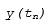using the solution at the immediately preceding time point,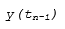, and the values of the derivative at multiple points between tn and tn-1. These points are minor steps.

Multistep solvers use the results at several preceding time steps to compute the current solution. Simulink provides one explicit multistep solver, `ode113`, and one implicit multistep solver, `ode15s`. Both are variable-step solvers.

#### Single-Order Versus Variable-Order Continuous Solvers

This distinction is based on the number of orders that the solver uses to solve the system of equation. Two variable-order solvers, `ode15s` and `ode113`, are part of the solver library. They use multiple orders to solve the system of equations. Specifically, the implicit, variable-step `ode15s` solver uses first-order through fifth-order equations, while the explicit, variable-step `ode113` solver uses first-order through thirteenth-order equations. For `ode15s`, you can limit the highest order applied via the `Maximum Order` parameter. For more information, see Maximum Order.

### Choose a Fixed-Step Solver

#### Fixed-Step Discrete Solver

The fixed-step discrete solver computes the time of the next simulation step by adding a fixed step size to the current time. The accuracy and the length of time of the resulting simulation depends on the size of the steps taken by the simulation: the smaller the step size, the more accurate the results are but the longer the simulation takes. By default, Simulink chooses the step size or you can choose the step size yourself. If you choose the default setting of `auto`, and if the model has discrete sample times, then Simulink sets the step size to the fundamental sample time of the model. Otherwise, if no discrete rates exist, Simulink sets the size to the result of dividing the difference between the simulation start and stop times by 50.

### Note

If you try to use the fixed-step discrete solver to update or simulate a model that has continuous states, an error message appears. Thus, selecting a fixed-step solver and then updating or simulating a model is a quick way to determine whether the model has continuous states.

#### Fixed-Step Continuous Solvers

The fixed-step continuous solvers, like the fixed-step discrete solver, compute the next simulation time by adding a fixed-size time step to the current time. For each of these steps, the continuous solvers use numerical integration to compute the values of the continuous states for the model. These values are calculated using the continuous states at the previous time step and the state derivatives at intermediate points (minor steps) between the current and the previous time step.

### Note

Simulink uses the fixed-step discrete solver for a model that contains no states or only discrete states, even if you specify a fixed-step continuous solver for the model.

Simulink provides two types of fixed-step continuous solvers — explicit and implicit.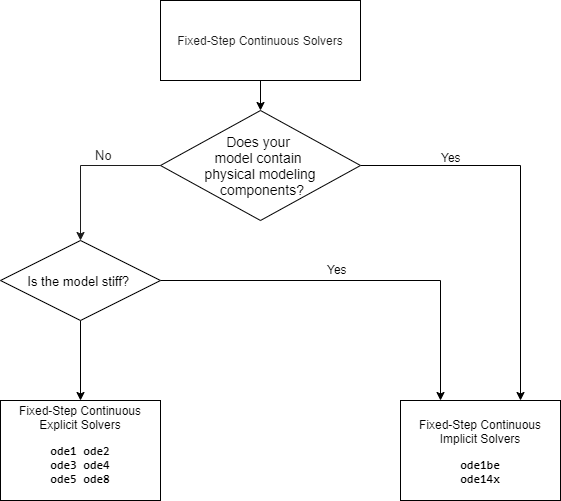The difference between these two types lies in the speed and the stability. An implicit solver requires more computation per step than an explicit solver but is more stable. Therefore, the implicit fixed-step solver that Simulink provides is more adept at solving a stiff system than the fixed-step explicit solvers. For more information, see Explicit Versus Implicit Continuous Solvers.

Fixed-Step Continuous Explicit Solvers.  Explicit solvers compute the value of a state at the next time step as an explicit function of the current values of both the state and the state derivative. A fixed-step explicit solver is expressed mathematically as:

`$x\left(n+1\right)=x\left(n\right)+h\ast Dx\left(n\right)$`

where x is the state, Dx is a solver-dependent function that estimates the state derivative, h is the step size, and n indicates the current time step.

Simulink provides a set of fixed-step continuous explicit solvers. The solvers differ in the specific numerical integration technique that they use to compute the state derivatives of the model. This table lists each solver and the integration technique it uses. The table lists the solvers in order of the computational complexity of the integration methods they use, from the least complex (`ode1`) to the most complex (`ode8`).

SolverIntegration TechniqueOrder of Accuracy

`ode1`

Euler's Method

First

`ode2`

Heun's Method

Second

`ode3`

Bogacki-Shampine Formula

Third

`ode4`

Fourth-Order Runge-Kutta (RK4) Formula

Fourth

`ode5`

Dormand-Prince (RK5) Formula

Fifth

`ode8`

Dormand-Prince RK8(7) Formula

Eighth

None of these solvers has an error control mechanism. Therefore, the accuracy and the duration of a simulation depend directly on the size of the steps taken by the solver. As you decrease the step size, the results become more accurate, but the simulation takes longer. Also, for any given step size, the higher the order of the solver, the more accurate the simulation results.

If you specify a fixed-step solver type for a model, then by default, Simulink selects the `FixedStepAuto` solver. Auto solver then selects an appropriate fixed-step solver that can handle both continuous and discrete states with moderate computational effort. As with the discrete solver, if the model has discrete rates (sample times), then Simulink sets the step size to the fundamental sample time of the model by default. If the model has no discrete rates, Simulink automatically uses the result of dividing the simulation total duration by 50. Consequently, the solver takes a step at each simulation time at which Simulink must update the discrete states of the model at its specified sample rates. However, it does not guarantee that the default solver accurately computes the continuous states of a model. Therefore, you may need to choose another solver, a different fixed step size, or both to achieve acceptable accuracy and an acceptable simulation time.

Fixed-Step Continuous Implicit Solvers.  An implicit solver computes the state at the next time step as an implicit function of the state at the current time step and the state derivative at the next time step. In other words:

`$x\left(n+1\right)-x\left(n\right)-h\ast Dx\left(n+1\right)=0$`

Simulink provides one fixed-step implicit solver: `ode14x`. This solver uses a combination of Newton's method and extrapolation from the current value to compute the value of a state at the next time step. You can specify the number of Newton's method iterations and the extrapolation order that the solver uses to compute the next value of a model state (see Fixed-step size (fundamental sample time)). The more iterations and the higher the extrapolation order that you select, the greater the accuracy you obtain. However, you simultaneously create a greater computational burden per step size.

#### How to Choose a Fixed-Step Continuous Solver

Any of the fixed-step continuous solvers in the Simulink product can simulate a model to any desired level of accuracy, given a small enough step size. Unfortunately, it is not possible or practical to decide without trial, the combination of solver and step size that will yield acceptable results for the continuous states in the shortest time. Determining the best solver for a particular model generally requires experimentation.

To select a fixed-step continuous solver,

1. Choose error tolerances. For more information, see Error Tolerances for Variable-Step Solvers.

2. Use one of the variable-step solvers to simulate your model to the level of accuracy that you want. Start with `ode45`. If your model runs slowly, your problem may be stiff and need an implicit solver. The results of this step give a good approximation of the correct simulation results and the appropriate fixed step size.

3. Use `ode1` to simulate your model at the default step size for your model. Compare the simulation results for `ode1` with the simulation for the variable-step solver. If the results are the same for the specified level of accuracy, you have found the best fixed-step solver for your model, namely `ode1`. You arrive at this conclusion because `ode1` is the simplest of the fixed-step solvers and hence yields the shortest simulation time for the current step size.

4. If `ode1` does not give satisfactory results, repeat the preceding steps with the other fixed-step solvers until you find one that gives accurate results with the least computational effort. The most efficient way to perform this task is to use a binary search technique:

1. Try `ode3`.

2. If `ode3` gives accurate results, try `ode2`. If `ode2` gives accurate results, it is the best solver for your model; otherwise, `ode3` is the best.

3. If `ode3` does not give accurate results, try `ode5`. If `ode5` gives accurate results, try `ode4`. If `ode4` gives accurate results, select it as the solver for your model; otherwise, select `ode5`.

4. If `ode5` does not give accurate results, reduce the simulation step size and repeat the preceding process. Continue in this way until you find a solver that solves your model accurately with the least computational effort.

### Choose a Variable-Step Solver

When you set the Type control of the Solver configuration pane to `Variable-step`, the Solver control allows you to choose one of the variable-step solvers. As with fixed-step solvers, the set of variable-step solvers comprises a discrete solver and a subset of continuous solvers. However, unlike the fixed-step solvers, the step size varies dynamically based on the local error.

The choice between the two types of variable-step solvers depends on whether the blocks in your model define states and, if so, the type of states that they define. If your model defines no states or defines only discrete states, select the discrete solver. If a model has no states or only discrete states, Simulink uses the discrete solver to simulate the model even if you specify a continuous solver. If the model has continuous states, the continuous solvers use numerical integration to compute the values of the continuous states at the next time step.

#### Variable-Step Continuous Solvers

Variable-step solvers dynamically vary the step size during the simulation. Each of these solvers increases or reduces the step size using its local error control to achieve the tolerances that you specify. Computing the step size at each time step adds to the computational overhead. However, it can reduce the total number of steps, and the simulation time required to maintain a specified level of accuracy.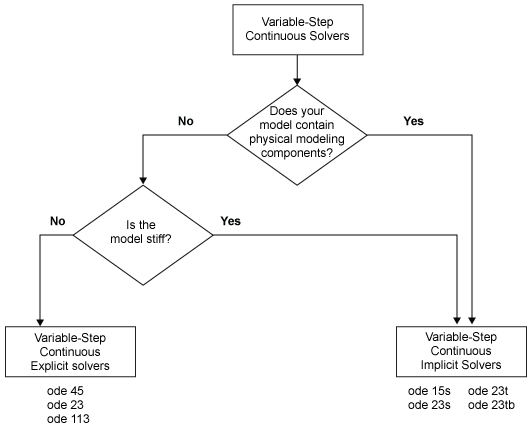You can further categorize the variable-step continuous solvers as: one-step or multistep, single-order or variable-order, and explicit or implicit. (See Compare Solvers for more information.)

#### Variable-Step Continuous Explicit Solvers

The variable-step explicit solvers are designed for nonstiff problems. Simulink provides four such solvers:

• `ode45`

• `ode23`

• `ode113`

• `odeN`

ODE SolverOne-Step MethodMultistep MethodOrder of AccuracyMethod
`ode45`X MediumRunge-Kutta, Dormand-Prince (4,5) pair
`ode23`X LowRunge-Kutta (2,3) pair of Bogacki & Shampine
`ode113` XVariable, Low to HighPECE Implementation of Adams-Bashforth-Moulton
`odeN`X Refer to Order of Accuracy in Fixed-Step Continuous Explicit SolversRefer to Integration Technique in Fixed-Step Continuous Explicit Solvers

ODE SolverTips on When to Use
ode45

In general, the `ode45` solver is the best to apply as a first try for most problems. This Runge-Kutta (4,5) solver is a fifth-order method that performs a fourth-order estimate of the error. This solver also uses a fourth-order “free” interpolant, which allows for event location and smoother plots.

If the `ode45` is computationally slow, your problem may be stiff and thus in need of an implicit solver.

ode23

The `ode23` can be more efficient than the `ode45` solver at crude error tolerances and in the presence of mild stiffness. This solver provides accurate solutions for “free” by applying a cubic Hermite interpolation to the values and slopes computed at the ends of a step.

ode113

For problems with stringent error tolerances or for computationally intensive problems, the Adams-Bashforth-Moulton PECE solver can be more efficient than `ode45`.

odeNThe `odeN` solver uses a nonadaptive Runge-Kutta integration whose order is determined by the Solver order parameter. `odeN` uses a fixed step size determined by the Max step size parameter, but the step size can be reduced to capture certain solver events, such as zero-crossings and discrete sample hits..

### Note

A good heuristic for choosing the `odeN` solver when simulation speed is important:

• The model contains lots of zero-crossings and/or solver resets

• The Solver Profiler does not detect any failed steps when profiling the model

#### Variable-Step Continuous Implicit Solvers

If your problem is stiff, try using one of the variable-step implicit solvers:

• `ode15s`

• `ode23s`

• `ode23t`

• `ode23tb`

ODE SolverOne-Step MethodMultistep MethodOrder of AccuracySolver Reset MethodMax. OrderMethod
`ode15s` XVariable, Low to MediumXXNumerical Differentiation Formulas (NDFs)
`ode23s`X Low  Second-order, modified Rosenbrock formula
`ode23t`X LowX Trapezoidal rule using a “free” interpolant
`ode23tb`X LowX TR-BDF2

Solver Reset Method.  For three of the solvers for stiff problems — `ode15s`, `ode23t`, and `ode23tb`— a drop-down menu for the Solver reset method appears on the Solver details section of the Configuration pane. This parameter controls how the solver treats a reset caused, for example, by a zero-crossing detection. The options allowed are `Fast` and `Robust`. `Fast` specifies that the solver does not recompute the Jacobian for a solver reset, whereas `Robust` specifies that the solver does. Consequently, the `Fast` setting is computationally faster but it may use a small step size in certain cases. To test for such cases, run the simulation with each setting and compare the results. If there is no difference in the results, you can safely use the `Fast` setting and save time. If the results differ significantly, try reducing the step size for the fast simulation.

Maximum Order.  For the `ode15s` solver, you can choose the maximum order of the numerical differentiation formulas (NDFs) that the solver applies. Since the `ode15s` uses first- through fifth-order formulas, the `Maximum order` parameter allows you to choose 1 through 5. For a stiff problem, you may want to start with order 2.

Tips for Choosing a Variable-Step Implicit Solver.  The following table provides tips relating to the application of variable-step implicit solvers. For an example comparing the behavior of these solvers, see sldemo_solvers.

ODE SolverTips on When to Use
ode15s

`ode15s` is a variable-order solver based on the numerical differentiation formulas (NDFs). NDFs are related to, but are more efficient than the backward differentiation formulas (BDFs), which are also known as Gear's method. The `ode15s` solver numerically generates the Jacobian matrices. If you suspect that a problem is stiff, or if `ode45` failed or was highly inefficient, try `ode15s`. As a rule, start by limiting the maximum order of the NDFs to 2.

ode23s

`ode23s` is based on a modified Rosenbrock formula of order 2. Because it is a one-step solver, it can be more efficient than `ode15s` at crude tolerances. Like `ode15s`, `ode23s` numerically generates the Jacobian matrix for you. However, it can solve certain kinds of stiff problems for which `ode15s` is not effective.

ode23t

The `ode23t` solver is an implementation of the trapezoidal rule using a “free” interpolant. Use this solver if your model is only moderately stiff and you need a solution without numerical damping. (Energy is not dissipated when you model oscillatory motion.)

ode23tb

`ode23tb` is an implementation of TR-BDF2, an implicit Runge-Kutta formula with two stages. The first stage is a trapezoidal rule step while the second stage uses a backward differentiation formula of order 2. By construction, the method uses the same iteration matrix in evaluating both stages. Like `ode23s`, this solver can be more efficient than `ode15s` at crude tolerances.

### Note

For a stiff problem, solutions can change on a time scale that is very small as compared to the interval of integration, while the solution of interest changes on a much longer time scale. Methods that are not designed for stiff problems are ineffective on intervals where the solution changes slowly because these methods use time steps small enough to resolve the fastest possible change. For more information, see Shampine, L. F., Numerical Solution of Ordinary Differential Equations, Chapman & Hall, 1994.

#### Support for Zero-Crossing Detection

The variable-step discrete and continuous solvers use zero-crossing detection (see Zero-Crossing Detection) to handle continuous signals.

#### Error Tolerances for Variable-Step Solvers

Local Error.  The variable-step solvers use standard control techniques to monitor the local error at each time step. During each time step, the solvers compute the state values at the end of the step and determine the local error—the estimated error of these state values. They then compare the local error to the acceptable error, which is a function of both the relative tolerance (rtol) and the absolute tolerance (atol). If the local error is greater than the acceptable error for any one state, the solver reduces the step size and tries again.

• The Relative tolerance measures the error relative to the size of each state. The relative tolerance represents a percentage of the state value. The default, 1e-3, means that the computed state is accurate to within 0.1%.

• Absolute tolerance is a threshold error value. This tolerance represents the acceptable error as the value of the measured state approaches zero.

The solvers require the error for the `i`th state, ei, to satisfy:

`${e}_{i}\le \mathrm{max}\left(rtol×|{x}_{i}|,ato{l}_{i}\right).$`

The following figure shows a plot of a state and the regions in which the relative tolerance and the absolute tolerance determine the acceptable error.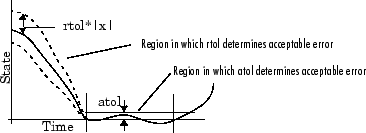Absolute Tolerances.  Your model has a global absolute tolerance that you can set on the Solver pane of the Configuration Parameters dialog box. This tolerance applies to all states in the model. You can specify `auto` or a real scalar. If you specify `auto` (the default), Simulink initially sets the absolute tolerance for each state based on the relative tolerance. If the relative tolerance is larger 1e-3, `abstol` is initialized at 1e-6. However, for `reltol` smaller than 1e-3, `abstol` for the state is initialized at `reltol * 1e-3`. As the simulation progresses, the absolute tolerance for each state resets to the maximum value that the state has assumed so far, times the relative tolerance for that state. Thus, if a state changes from 0 to 1 and `reltol` is 1e-3, `abstol` initializes at 1e-6 and by the end of the simulation reaches 1e-3 also. If a state goes from 0 to 1000, then `abstol` changes to 1.

Now, if the state changes from 0 to 1 and `reltol` is set at 1e-4, then `abstol`initializes at 1e-7 and by the end of the simulation reaches a value of 1e-4.

If the computed initial value for the absolute tolerance is not suitable, you can determine an appropriate value yourself. You might have to run a simulation more than once to determine an appropriate value for the absolute tolerance. You can also specify if the absolute tolerance should adapt similar to its `auto` setting by enabling or disabling the `AutoScaleAbsTol` parameter. For more information, see Auto scale absolute tolerance.

Several blocks allow you to specify absolute tolerance values for solving the model states that they compute or that determine their output:

The absolute tolerance values that you specify for these blocks override the global settings in the Configuration Parameters dialog box. You might want to override the global setting if, for example, the global setting does not provide sufficient error control for all of your model states because they vary widely in magnitude. You can set the block absolute tolerance to:

• `auto`

• `1` (same as` auto`)

• `positive scalar`

• `real vector` (having a dimension equal to the number of corresponding continuous states in the block)

Tips.  If you do choose to set the absolute tolerance, keep in mind that too low of a value causes the solver to take too many steps in the vicinity of near-zero state values. As a result, the simulation is slower.

On the other hand, if you set the absolute tolerance too high, your results can be inaccurate as one or more continuous states in your model approach zero.

Once the simulation is complete, you can verify the accuracy of your results by reducing the absolute tolerance and running the simulation again. If the results of these two simulations are satisfactorily close, then you can feel confident about their accuracy.

### Choose a Jacobian Method for an Implicit Solver

#### The Solver Jacobian

For implicit solvers, Simulink must compute the solver Jacobian, which is a submatrix of the Jacobian matrix associated with the continuous representation of a Simulink model. In general, this continuous representation is of the form:

`$\begin{array}{l}\stackrel{˙}{x}=f\left(x,t,u\right)\\ y=g\left(x,t,u\right).\end{array}$`

The Jacobian, J, formed from this system of equations is:

`$J=\left(\begin{array}{cc}\frac{\partial f}{\partial x}& \frac{\partial f}{\partial u}\\ \frac{\partial g}{\partial x}& \frac{\partial g}{\partial u}\end{array}\right)=\left(\begin{array}{cc}A& B\\ C& D\end{array}\right).$`

In turn, the solver Jacobian is the submatrix, ${J}_{x}$.

`${J}_{x}=A=\frac{\partial f}{\partial x}.$`

Sparsity of Jacobian.  For many physical systems, the solver Jacobian Jx is sparse, meaning that many of the elements of Jx are zero.

Consider the following system of equations:

`$\begin{array}{l}{\stackrel{˙}{x}}_{1}={f}_{1}\left({x}_{1},{x}_{3}\right)\\ {\stackrel{˙}{x}}_{2}={f}_{2}\left({x}_{2}\right)\\ {\stackrel{˙}{x}}_{3}={f}_{3}\left({x}_{2}\right).\end{array}$`

From this system, you can derive a sparsity pattern that reflects the structure of the equations. The pattern, a Boolean matrix, has a 1 for each${x}_{i}$ that appears explicitly on the right-hand side of an equation. Therefore, you attain:

`${J}_{x,pattern}=\left(\begin{array}{ccc}1& 0& 1\\ 0& 1& 0\\ 0& 1& 0\end{array}\right)$`

As discussed in Full and Sparse Perturbation Methods and Full and Sparse Analytical Methods respectively, the Sparse Perturbation Method and the Sparse Analytical Method may be able to take advantage of this sparsity pattern to reduce the number of computations necessary and improve performance.

#### Solver Jacobian Methods

When you choose an implicit solver from the Solver pane of the configuration parameters dialog box, a parameter called ```Solver Jacobian method``` and a drop-down menu appear. This menu has five options for computing the solver Jacobian:

• auto

• Sparse perturbation

• Full perturbation

• Sparse analytical

• Full analytical### Note

If you set Automatic solver parameter selection to `error` in the Solver Diagnostics pane, and you choose a different solver method than Simulink, you may receive an error.

Limitations.  The solver Jacobian methods have the following limitations associated with them.

• If you select an analytical Jacobian method, but one or more blocks in the model do not have an analytical Jacobian, then Simulink applies a perturbation method.

• If you select sparse perturbation and your model contains data store blocks, Simulink applies the full perturbation method.

#### Heuristic 'auto' Method

The default setting for the `Solver Jacobian method` is `auto`. Selecting this choice causes Simulink to perform a heuristic to determine which of the remaining four methods best suits your model. This algorithm is depicted in the following flow chart.Because sparse methods are beneficial for models having a large number of states, if 50 or more states exist in your model, the heuristic chooses a sparse method. The logic also leads to a sparse method if you specify `ode23s` because, unlike other implicit solvers, `ode23s` generates a new Jacobian at every time step. A sparse analytical or a sparse perturbation method is, therefore, highly advantageous. The heuristic also ensures that the analytical methods are used only if every block in your model can generate an analytical Jacobian.

#### Full and Sparse Perturbation Methods

The full perturbation method was the standard numerical method that Simulink used to solve a system. For this method, Simulink solves the full set of perturbation equations and uses LAPACK for linear algebraic operations. This method is costly from a computational standpoint, but it remains the recommended method for establishing baseline results.

The sparse perturbation method attempts to improve the run-time performance by taking mathematical advantage of the sparse Jacobian pattern. Returning to the sample system of three equations and three states,

`$\begin{array}{l}{\stackrel{˙}{x}}_{1}={f}_{1}\left({x}_{1},{x}_{3}\right)\\ {\stackrel{˙}{x}}_{2}={f}_{2}\left({x}_{2}\right)\\ {\stackrel{˙}{x}}_{3}={f}_{3}\left({x}_{2}\right).\end{array}$`

The solver Jacobian is:

 `$\begin{array}{c}{J}_{x}=\left(\begin{array}{ccc}\frac{\partial {f}_{1}}{\partial {x}_{1}}& \frac{\partial {f}_{1}}{\partial {x}_{2}}& \frac{\partial {f}_{1}}{\partial {x}_{3}}\\ \frac{\partial {f}_{2}}{\partial {x}_{1}}& \frac{\partial {f}_{2}}{\partial {x}_{2}}& \frac{\partial {f}_{2}}{\partial {x}_{3}}\\ \frac{\partial {f}_{3}}{\partial {x}_{1}}& \frac{\partial {f}_{3}}{\partial {x}_{2}}& \frac{\partial {f}_{3}}{\partial {x}_{3}}\end{array}\right)\\ =\left(\begin{array}{ccc}\frac{{f}_{1}\left({x}_{1}+\Delta {x}_{1},{x}_{2},{x}_{3}\right)-{f}_{1}}{\Delta {x}_{1}}& \frac{{f}_{1}\left({x}_{1},{x}_{2}+\Delta {x}_{2},{x}_{3}\right)-{f}_{1}}{\Delta {x}_{2}}& \frac{{f}_{1}\left({x}_{1},{x}_{2},{x}_{3}+\Delta {x}_{3}\right)-{f}_{1}}{\Delta {x}_{3}}\\ \frac{{f}_{2}\left({x}_{1}+\Delta {x}_{1},{x}_{2},{x}_{3}\right)-{f}_{2}}{\Delta {x}_{1}}& \frac{{f}_{2}\left({x}_{1},{x}_{2}+\Delta {x}_{2},{x}_{3}\right)-{f}_{2}}{\Delta {x}_{2}}& \frac{{f}_{2}\left({x}_{1},{x}_{2},{x}_{3}+\Delta {x}_{3}\right)-{f}_{2}}{\Delta {x}_{3}}\\ \frac{{f}_{3}\left({x}_{1}+\Delta {x}_{1},{x}_{2},{x}_{3}\right)-{f}_{3}}{\Delta {x}_{1}}& \frac{{f}_{3}\left({x}_{1},{x}_{2}+\Delta {x}_{2},{x}_{3}\right)-{f}_{3}}{\Delta {x}_{2}}& \frac{{f}_{3}\left({x}_{1},{x}_{2},{x}_{3}+\Delta {x}_{3}\right)-{f}_{3}}{\Delta {x}_{3}}\end{array}\right)\end{array}$`

It is, therefore, necessary to perturb each of the three states three times and to evaluate the derivative function three times. For a system with n states, this method perturbs the states n times.

By applying the sparsity pattern and perturbing states x1 and x 2 together, this matrix reduces to:

 `${J}_{x}=\left(\begin{array}{ccc}\frac{{f}_{1}\left({x}_{1}+\Delta {x}_{1},{x}_{2}+\Delta {x}_{2},{x}_{3}\right)-{f}_{1}}{\Delta {x}_{1}}& 0& \frac{{f}_{1}\left({x}_{1},{x}_{2},{x}_{3}+\Delta {x}_{3}\right)-{f}_{1}}{\Delta {x}_{3}}\\ 0& \frac{{f}_{2}\left({x}_{1}+\Delta {x}_{1},{x}_{2}+\Delta {x}_{2},{x}_{3}\right)-{f}_{2}}{\Delta {x}_{2}}& 0\\ 0& \frac{{f}_{3}\left({x}_{1}+\Delta {x}_{1},{x}_{2}+\Delta {x}_{2},{x}_{3}\right)-{f}_{3}}{\Delta {x}_{2}}& 0\end{array}\right)$`

The solver can now solve columns 1 and 2 in one sweep. While the sparse perturbation method saves significant computation, it also adds overhead to compilation. It might even slow down the simulation if the system does not have a large number of continuous states. A tipping point exists for which you obtain increased performance by applying this method. In general, systems having a large number of continuous states are usually sparse and benefit from the sparse method.

The sparse perturbation method, like the sparse analytical method, uses UMFPACK to perform linear algebraic operations. Also, the sparse perturbation method supports both RSim and Rapid Accelerator mode.

#### Full and Sparse Analytical Methods

The full and sparse analytical methods attempt to improve performance by calculating the Jacobian using analytical equations rather than the perturbation equations. The sparse analytical method, also uses the sparsity information to accelerate the linear algebraic operations required to solve the ordinary differential equations.

#### Sparsity Pattern

For details on how to access and interpret the sparsity pattern in MATLAB®, see sldemo_metro.

#### Support for Code Generation

While the sparse perturbation method supports RSim, the sparse analytical method does not. Consequently, regardless of which sparse method you select, any generated code uses the sparse perturbation method. This limitation applies to Rapid Accelerator mode as well.